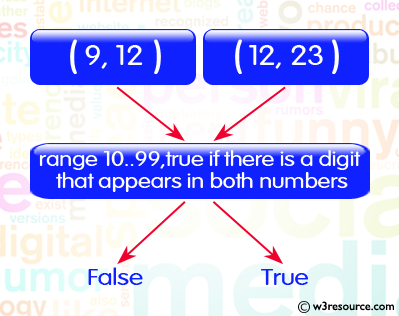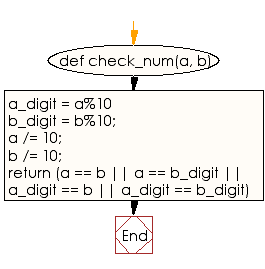﻿ Ruby Basic exercises: Check two given integers, each in the range 10..99, return true if there is a digit that appears in both numbers - w3resource# Ruby Basic Exercises: Check two given integers, each in the range 10..99, return true if there is a digit that appears in both numbers

## Ruby Basic: Exercise-51 with Solution

Write a Ruby program to check two given integers, each in the range 10..99, return true if there is a digit that appears in both numbers.Ruby Code:

``````def check_num(a, b)
a_digit = a%10
b_digit = b%10;
a /= 10;
b /= 10;
return (a == b || a == b_digit || a_digit == b || a_digit == b_digit)
end
print check_num(9, 12),"\n"
print check_num(15, 51),"\n"
print check_num(12, 23)
``````

Output:

```false
true
true
```

Flowchart:Ruby Code Editor: